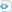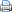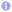# MPL – Collective communication

In a previous post I gave a very short introduction to the Message Passing Library (MPL), which is a C++ message passing library based on MPI. Although it does not provide a direct mapping of the C API to C++ it comes with all modes of collective communication as defined be the MPI standard, e.g., barrier, broadcast, gather, scatter, reduce and so on. The following program does not perform any meaningful calculation, but illustrates some modes of collective communication.

 Collective communication with MPL.#include <cstdlib>
#include <complex>
#include <iostream>
#include <vector>
#include <mpl/mpl.hpp>

int main() {
const mpl::communicator &comm_world=mpl::environment::comm_world();
std::vector<int> v;
if (comm_world.rank()==0)
for (int i=0; i<comm_world.size(); ++i)
v.push_back(i);
int x;
// rank 0 scatters data to all processes
comm_world.scatter(0, v.data(), x);
std::cout << "rank " << comm_world.rank() << " got " << x << '\n';
// wait until all processes have reached this point
comm_world.barrier();
x*=2;
// rank 0 gathers data from all processes
comm_world.gather(0, x, v.data());
if (comm_world.rank()==0)
for (int i=0; i<comm_world.size(); ++i)
std::cout << "got " << v[i] << " from rank " << i << '\n';
// wait until all processes have reached this point
comm_world.barrier();
// calculate global sum and pass result to rank 0
if (comm_world.rank()==0) {
int sum;
comm_world.reduce(mpl::plus<int>(), 0, x, sum);
std::cout << "sum = " << sum << '\n';
} else
comm_world.reduce(mpl::plus<int>(), 0, x);
// wait until all processes have reached this point
comm_world.barrier();
// calculate global sum and pass result to all
comm_world.allreduce(mpl::plus<int>(), x);
std::cout << "sum = " << x << '\n';
return EXIT_SUCCESS;
}

Note that the reduction operation (addition) in the example above is specified as an anonymous function object. In addition to addition, MPL provides multiplication, logical operations »and« and »or«, bitwise operations »and«, »or«, and »xor« as well as minimum and maximum. A reduction operation must take two arguments of the same kind and produce a result of the same type as the arguments. With MPL it becomes very easy to define custom reduction operations, as the following example shows. Note that it is required, that the reduction operation is implemented by a class, which is derived from std::function and has no member variables.

 Custom reduction operations.#include <cstdlib>
#include <ctime>
#include <iostream>
#include <functional>
#include <vector>
#include <mpl/mpl.hpp>

// calculate least common multiple of two arguments
template<typename T>
class lcm : public std::function<T (T, T)> {
// helper: calculate greatest common divisor
T gcd(T a, T b) {
T zero=T(), t;
if (a<zero) a=-a;
if (b<zero) b=-b;
while (b>zero) {
t=a%b;  a=b;  b=t;
}
return a;
}
public:
T operator()(T a, T b) {
T zero=T();
T t((a/gcd(a, b))*b);
if (t<zero)
return -t;
return t;
}
};

int main() {
const mpl::communicator &comm_world=mpl::environment::comm_world();
// generate data
std::srand(std::time(0)*comm_world.rank());  // random seed
int v=std::rand()%12+1;
// calculate least common multiple and send result to rank 0
if (comm_world.rank()==0) {
int result;
// calculate least common multiple
comm_world.reduce(lcm<int>(), 0, v, result);
// display data from all ranks
std::cout << "Arguments:\n";
for (int r=0; r<comm_world.size(); ++r) {
if (r>0)
comm_world.recv(v, r);
std::cout << v << '\n';
}
// display results of global reduction
std::cout << "\nResult:\n";
std::cout << result << '\n';
} else {
// calculate least common multiple
comm_world.reduce(lcm<int>(), 0, v);
// send data to rank 0 for display
comm_world.send(v, 0);
}
return EXIT_SUCCESS;
}
MPI provides reductions operations MINLOC and MAXLOC, which simultaneously determine the minimum or maximum plus the location of the minimum or maximum. In MPL, however, such specific operations are not needed. Minimum and maximum operations for pairs of some scalar type and an integer do the same job as the following example shows.
 Simultaneous determination of minimum and its location.#include <cstdlib>
#include <ctime>
#include <iostream>
#include <iomanip>
#include <vector>
#include <utility>
#include <mpl/mpl.hpp>

typedef std::pair<double, int> pair_t;

int main() {
const mpl::communicator &comm_world=mpl::environment::comm_world();
// generate data
std::srand(std::time(0)*comm_world.rank());  // random seed
const int n=8;
std::vector<pair_t> v(n);
for (pair_t &i : v)
i=std::make_pair(static_cast<double>(std::rand())/RAND_MAX, comm_world.rank());
// calculate minium and its location and send result to rank 0
mpl::contiguous_layout<pair_t> layout(n);
if (comm_world.rank()==0) {
std::vector<pair_t> result(n);
// calculate minimum
comm_world.reduce(mpl::min<pair_t>(), 0, v.data(), result.data(), layout);
// display data from all ranks
std::cout << "Arguments:\n";
for (int r=0; r<comm_world.size(); ++r) {
if (r>0)
comm_world.recv(v.data(), layout, r);
for (pair_t i : v)
std::cout << std::fixed << std::setprecision(5) << i.first << ' ' << i.second << '\t';
std::cout << '\n';
}
// display results of global reduction
std::cout << "\nResults:\n";
for (pair_t i : result)
std::cout << std::fixed << std::setprecision(5) << i.first << ' ' << i.second << '\t';
std::cout << '\n';
} else {
// calculate minium and its location and send result to rank 0
comm_world.reduce(mpl::min<pair_t>(), 0, v.data(), layout);
// send data to rank 0 for display
comm_world.send(v.data(), layout, 0);
}
return EXIT_SUCCESS;
}